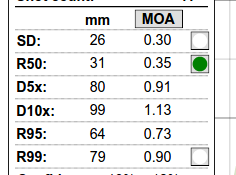# 4.2. Measures of precision

As mentioned here and here, a single group's extreme spread as a measure of rifle precision is both scientifically invalid and practically useless.

Rifle precision can, however, be rather accurately described by known statistical models. TARAN uses the Rayleigh distribution model to calculate precision parameters. The outcome of the modelling is presented in the left-hand pane:* SD (a.k.a. sigma) is the normal distribution's standard deviation (and Rayleigh's scale parameter).
* R50, also known as Circular Error Probable (CEP) or Mean Radius, is the radius, which would statistically contain 50% of all shots -- a very common ballistic and military measure of a weapon's precision. A caveat on this one: it is a result of a mathematical model, and represents what would happen with an infinite number of shots; the actual shots accounted for in the project may be distributed slightly differently, and not exactly fall 50/50 inside/outside of R50 (but with enough shots it should be very close).
* D5x and D10x, calculated mostly for entertainment purposes, represent the average extreme spread of 5-shot and 10-shot groups that can be expected from the rifle. Nota Bene: it does not mean that shots of such groups will be contained in a given diameter circle around the average PoI; actual centres of groups may be very different from the real rifle's PoI and it's mathematical estimation.
* R95 and R99 -- respectively radii containing 95% and 99% of the shots. R95 is a good indication of a "likely hit" probability.

You can toggle the display (thin grey circles) of SD, R50 and R99 by clicking on small buttons in the corresponding rows. On the example image above, R50 is displayed.

R95 is displayed as a thick green circle.